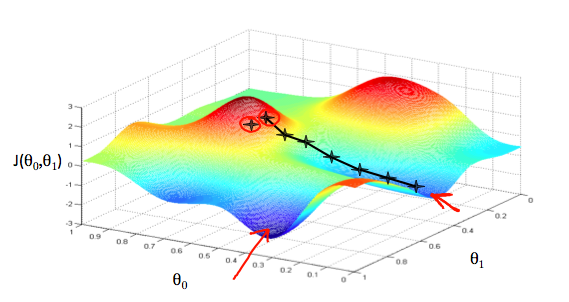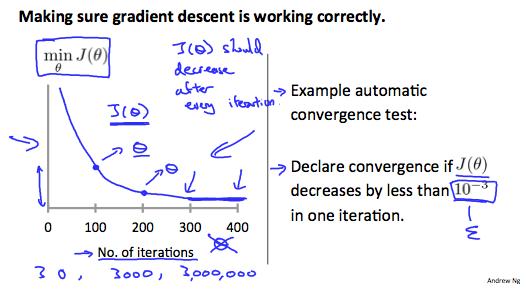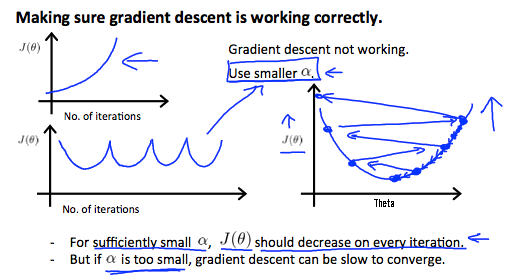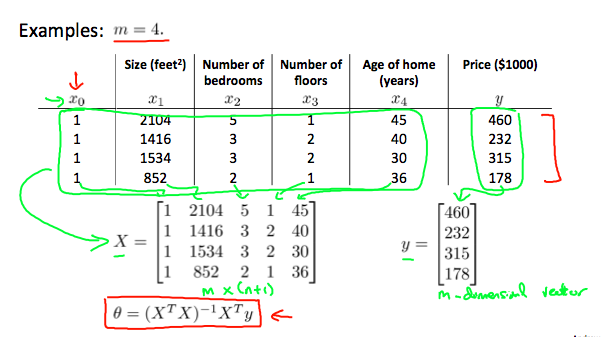# 线性回归

Size(x) Price(y)
2104 460
1035 224
868 230
642 126

### 二维梯度下降## 多维线性回归

Size(x1) #bed room (x2) #floors(x3) Price(y)
2104 5 2 460
1035 4 1 224
868 3 2 230
642 2 1 126

• $m$ 表示样本数
• $n$ 表示feature个数
• $x^{(i)}$ 表示第$i$组训练样本
• $x_j^{(i)}$ 表示第$i$个样本中的第$j$个feature

### 多维梯度下降

function [theta, J_history] = gradientDescentMulti(X, y, theta, alpha, num_iters)
%   taking num_iters gradient steps with learning rate alpha

% Initialize some useful values
m = length(y); % number of training examples
J_history = zeros(num_iters, 1);

for iter = 1:num_iters

num_features = size(X,2);
h = X*theta;

for j = 1:num_features
x = X(:,j);
theta(j) = theta(j) - alpha*(1/m)*sum((h-y).* x);
end

% ============================================================

% Save the cost J in every iteration
J_history(iter) = computeCostMulti(X, y, theta);

end

end


## Feature Scaling

Idea: Make sure features are on a similar scale.

E.g.:x1 = size(0-200 feet),x2=number of bedrooms(1-5)

x1 = size(0-200 feet)/5,x2=(number of bedrooms)/5,则 contour 图会变为接近圆形，梯度下降收敛的速度会加快。通常为了加速收敛，会将每个 feature 值(每个xi)统一到某个区间里，比如 $0\le {x}_{1}\le 3$$-2\le {x}_{2}\le 0.5$等等

## Mean normalization

Replace ${x}_{i}$with ${x}_{i}-{\mu }_{i}$ to make features have approximately zero mean.实际上就是将 feature 归一化，

• ${\mu }_{i}$

是所有 ${x}_{i}$

• ${\mu }_{i}$

xi的区间范围，(max-min)

Note that dividing by the range, or dividing by the standard deviation, give different results. The quizzes in this course use range - the programming exercises use standard deviation.

For example, if xi represents housing prices with a range of 100 to 2000 and a mean value of 1000, then

$x i := p r i c e − 1000 1900$
• μ表示所有 feature 的平均值
• s = max - min

## Learning Rate

$θ j := θ j - α ∂ ∂ θ j J ( θ )$• Summary: - if α is too small: slow convergence - if α is too large: J(θ)may not decrease on every iteration; may not converge - To choose α , try: …, 0.001, 0.003, 0.01,0.03, 0.1,0.3, 1, …

## Polynomial regression

$h θ ( x ) = θ 0 + θ 1 x 1 + θ 2 x 2 + θ 3 x 3 + ⋯ + θ n x n$

We can improve our features and the form of our hypothesis function in a couple different ways. We can combine multiple features into one. For example, we can combine x1 and x2 into a new feature x3 by taking x1⋅x2.

$h θ ( x ) = θ 0 + θ 1 x 1 + θ 2 x 1 2 or h θ ( x ) = θ 0 + θ 1 x 1 + θ 2 x 1 2 + θ 3 x 1 3$

## Normal Equation

$J ( θ ) = 1 2 m ∑ i = 1 m h θ ( x i ) − y i 2$

$∂∂θjJ(θ) = 0 (for every j)$

$X = 1 x1(1) x2(1) ... xn(1) 1 x1(2) x2(2) ... xn(2) 1 ... ... ... ... 1 x1(m) x2(m) ... xn(m) , θ = θ1 θ2 ... θn , Y = y1 y2 ... ym$

• $X$

$m\*\mathrm{\left(n+1\right)}$的矩阵

• $\theta$

$\mathrm{\left(n+1\right)}\*1$的矩阵

• $Y$

$m\*1$的矩阵• 单位矩阵 E，$\mathrm{AE}=\mathrm{EA}=A$
• 矩阵的逆${A}^{\mathrm{-1}}$，A 必须为方阵，$A{A}^{\mathrm{-1}}={A}^{\mathrm{-1}}A=E$

1. 先把 θ 左边的矩阵变成一个方阵。通过乘以${X}^{T}$可以实现，则有 ${X}^{T}X·\theta ={X}^{T}Y$

2. 把 θ 左边的部分变成一个单位矩阵，这样左边就只剩下 θ，$\left({X}^{T}X{\right)}^{-1}{X}^{T}X·\theta =\left({X}^{T}X{\right)}^{-1}{X}^{T}Y$

3. 由于$\left({X}^{T}X{\right)}^{-1}{X}^{T}X=E$，因此式子变为$\theta =\left({X}^{T}X{\right)}^{-1}{X}^{T}Y$，这就Normal Equation的表达式。

Need to choose alpha No need to choose alpha
Needs many iterations No need to iterate
$O\left(k{n}^{2}\right)$ $O\left({n}^{3}\right)$ need to calculate inverse of ${X}^{T}X$
Works well when n is large Slow if n is very large

When implementing the normal equation in octave we want to use the pinv function rather than inv. The pinv function will give you a value of θ even if${X}^{T}X$ is not invertible(不可逆).

If ${X}^{T}X$ is noninvertible, the common causes might be having :

• Redundant features, where two features are very closely related (i.e. they are linearly dependent)
• Too many features (e.g. m ≤ n). In this case, delete some features or use “regularization” (to be explained in a later lesson).

Solutions to the above problems include deleting a feature that is linearly dependent with another or deleting one or more features when there are too many features.